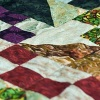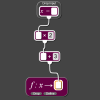# Resources tagged with: Factors and multiples

Filter by: Content type:
Age range:
Challenge level:

### There are 153 results

Broad Topics > Properties of Numbers > Factors and multiples### Flashing Lights

##### Age 7 to 11 Challenge Level:

Norrie sees two lights flash at the same time, then one of them flashes every 4th second, and the other flashes every 5th second. How many times do they flash together during a whole minute?### Give Me Four Clues

##### Age 7 to 11 Challenge Level:

Four of these clues are needed to find the chosen number on this grid and four are true but do nothing to help in finding the number. Can you sort out the clues and find the number?### Multiplication Series: Number Arrays

##### Age 5 to 11

This article for teachers describes how number arrays can be a useful representation for many number concepts.### Money Measure

##### Age 7 to 11 Challenge Level:

How can you use just one weighing to find out which box contains the lighter ten coins out of the ten boxes?### What's in the Box?

##### Age 7 to 11 Challenge Level:

This big box multiplies anything that goes inside it by the same number. If you know the numbers that come out, what multiplication might be going on in the box?### What Do You Need?

##### Age 7 to 11 Challenge Level:

Four of these clues are needed to find the chosen number on this grid and four are true but do nothing to help in finding the number. Can you sort out the clues and find the number?### Venn Diagrams

##### Age 5 to 11 Challenge Level:

How will you complete these Venn diagrams?### Times Right

##### Age 11 to 16 Challenge Level:

Using the digits 1, 2, 3, 4, 5, 6, 7 and 8, mulitply a two two digit numbers are multiplied to give a four digit number, so that the expression is correct. How many different solutions can you find?### What Two ...?

##### Age 7 to 11 Short Challenge Level:

56 406 is the product of two consecutive numbers. What are these two numbers?### Product Sudoku

##### Age 11 to 16 Challenge Level:

The clues for this Sudoku are the product of the numbers in adjacent squares.### Path to the Stars

##### Age 7 to 11 Challenge Level:

Is it possible to draw a 5-pointed star without taking your pencil off the paper? Is it possible to draw a 6-pointed star in the same way without taking your pen off?### Factoring Factorials

##### Age 11 to 14 Challenge Level:

Find the highest power of 11 that will divide into 1000! exactly.### Tom's Number

##### Age 7 to 11 Challenge Level:

Work out Tom's number from the answers he gives his friend. He will only answer 'yes' or 'no'.### The Remainders Game

##### Age 7 to 14 Challenge Level:

Play this game and see if you can figure out the computer's chosen number.### Thirty Six Exactly

##### Age 11 to 14 Challenge Level:

The number 12 = 2^2 � 3 has 6 factors. What is the smallest natural number with exactly 36 factors?### Spelling Circle

##### Age 7 to 11 Challenge Level:

Find the words hidden inside each of the circles by counting around a certain number of spaces to find each letter in turn.### Eminit

##### Age 11 to 14 Challenge Level:

The number 8888...88M9999...99 is divisible by 7 and it starts with the digit 8 repeated 50 times and ends with the digit 9 repeated 50 times. What is the value of the digit M?### American Billions

##### Age 11 to 14 Challenge Level:

Play the divisibility game to create numbers in which the first two digits make a number divisible by 2, the first three digits make a number divisible by 3...### Big Powers

##### Age 11 to 16 Challenge Level:

Three people chose this as a favourite problem. It is the sort of problem that needs thinking time - but once the connection is made it gives access to many similar ideas.### A First Product Sudoku

##### Age 11 to 14 Challenge Level:

Given the products of adjacent cells, can you complete this Sudoku?### Divide it Out

##### Age 7 to 11 Challenge Level:

What is the lowest number which always leaves a remainder of 1 when divided by each of the numbers from 2 to 10?### Ducking and Dividing

##### Age 7 to 11 Challenge Level:

Your vessel, the Starship Diophantus, has become damaged in deep space. Can you use your knowledge of times tables and some lightning reflexes to survive?### Powerful Factorial

##### Age 11 to 14 Challenge Level:

6! = 6 x 5 x 4 x 3 x 2 x 1. The highest power of 2 that divides exactly into 6! is 4 since (6!) / (2^4 ) = 45. What is the highest power of two that divides exactly into 100!?### Just Repeat

##### Age 11 to 14 Challenge Level:

Think of any three-digit number. Repeat the digits. The 6-digit number that you end up with is divisible by 91. Is this a coincidence?### Guess the Dominoes for Two

##### Age 7 to 11 Challenge Level:

Guess the Dominoes for child and adult. Work out which domino your partner has chosen by asking good questions.### Oh! Hidden Inside?

##### Age 11 to 14 Challenge Level:

Find the number which has 8 divisors, such that the product of the divisors is 331776.### Missing Multipliers

##### Age 7 to 14 Challenge Level:

What is the smallest number of answers you need to reveal in order to work out the missing headers?### Remainders

##### Age 7 to 14 Challenge Level:

I'm thinking of a number. My number is both a multiple of 5 and a multiple of 6. What could my number be?### Funny Factorisation

##### Age 11 to 16 Challenge Level:

Using the digits 1 to 9, the number 4396 can be written as the product of two numbers. Can you find the factors?### Multiples Sudoku

##### Age 11 to 14 Challenge Level:

Each clue in this Sudoku is the product of the two numbers in adjacent cells.### Alison's Quilt

##### Age 11 to 14 Challenge Level:

Nine squares are fitted together to form a rectangle. Can you find its dimensions?### Which Is Quicker?

##### Age 7 to 11 Challenge Level:

Which is quicker, counting up to 30 in ones or counting up to 300 in tens? Why?### Transposition Cipher

##### Age 11 to 16 Challenge Level:

Can you work out what size grid you need to read our secret message?### Inclusion Exclusion

##### Age 11 to 14 Challenge Level:

How many integers between 1 and 1200 are NOT multiples of any of the numbers 2, 3 or 5?### Guess the Dominoes

##### Age 7 to 11 Challenge Level:

This task depends on learners sharing reasoning, listening to opinions, reflecting and pulling ideas together.### Three Neighbours

##### Age 7 to 11 Challenge Level:

Look at three 'next door neighbours' amongst the counting numbers. Add them together. What do you notice?### Odds and Threes

##### Age 7 to 11 Challenge Level:

A game for 2 people using a pack of cards Turn over 2 cards and try to make an odd number or a multiple of 3.### Number Families

##### Age 11 to 14 Challenge Level:

How many different number families can you find?### Scoring with Dice

##### Age 7 to 11 Challenge Level:

I throw three dice and get 5, 3 and 2. Add the scores on the three dice. What do you get? Now multiply the scores. What do you notice?### Gabriel's Problem

##### Age 11 to 14 Challenge Level:

Gabriel multiplied together some numbers and then erased them. Can you figure out where each number was?### Satisfying Statements

##### Age 11 to 14 Challenge Level:

Can you find any two-digit numbers that satisfy all of these statements?### Number Tracks

##### Age 7 to 11 Challenge Level:

Ben’s class were cutting up number tracks. First they cut them into twos and added up the numbers on each piece. What patterns could they see?### Sieve of Eratosthenes

##### Age 11 to 14 Challenge Level:

Follow this recipe for sieving numbers and see what interesting patterns emerge.### Down to Nothing

##### Age 7 to 11 Challenge Level:

A game for 2 or more people. Starting with 100, subratct a number from 1 to 9 from the total. You score for making an odd number, a number ending in 0 or a multiple of 6.### Exploring Simple Mappings

##### Age 11 to 14 Challenge Level:

Explore the relationship between simple linear functions and their graphs.### Which Numbers? (1)

##### Age 7 to 11 Challenge Level:

I am thinking of three sets of numbers less than 101. They are the red set, the green set and the blue set. Can you find all the numbers in the sets from these clues?### Which Numbers? (2)

##### Age 7 to 11 Challenge Level:

I am thinking of three sets of numbers less than 101. Can you find all the numbers in each set from these clues?### Star Product Sudoku

##### Age 11 to 16 Challenge Level:

The puzzle can be solved by finding the values of the unknown digits (all indicated by asterisks) in the squares of the $9\times9$ grid.### Three Spinners

##### Age 7 to 11 Challenge Level:

These red, yellow and blue spinners were each spun 45 times in total. Can you work out which numbers are on each spinner?### Being Resilient - Primary Number

##### Age 5 to 11 Challenge Level:

Number problems at primary level that may require resilience.